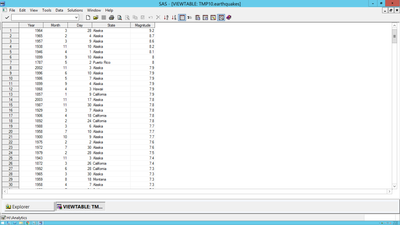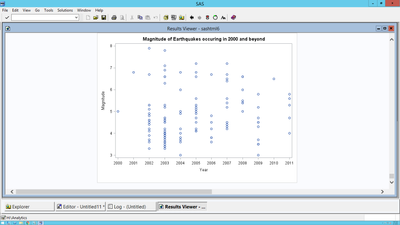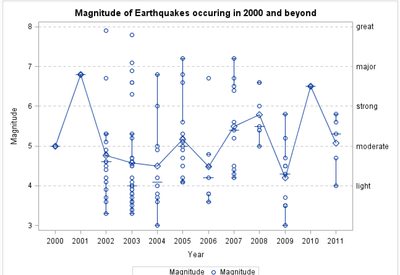## Overlaying the mean and other reference lines onto an existing graph

Hello,

I have an original data set that looks like this:Thus far I've created a scatter plot using this code:

```proc sgplot data=W2.earthquakes(where=(year >= 2000));
XAXIS type = discrete;
Scatter X = year Y = magnitude;
Title 'Magnitude of Earthquakes occuring in 2000 and beyond';
run;```

And I get a graph that looks like this:I want to overlay the mean magnitude for each year on the same graph in a red line. I also want to incorporate reference lines that indicate different levels of earthquakes. These reference lines  would be light, moderate, strong, major, and great earthquakes and they would be defined at magnitudes of 4.0, 5.0, 6.0, 7.0, and 8.0. The ideal final output would look like the graph above but with the mean line and the additional reference lines running through it.

I'm at a loss of how to do calculate the average within the sgplot proc. Do I need a create a new dataset with the average as a variable? Would it be possible to incorporate proc means within proc sgplot?

Thank you!

4 REPLIES 4

## Re: Overlaying the mean and other reference lines onto an existing graph

@Errant wrote:

Hello,

I have an original data set that looks like this:Thus far I've created a scatter plot using this code:

```proc sgplot data=W2.earthquakes(where=(year >= 2000));
XAXIS type = discrete;
Scatter X = year Y = magnitude;
Title 'Magnitude of Earthquakes occuring in 2000 and beyond';
run;```

And I get a graph that looks like this:I want to overlay the mean magnitude for each year on the same graph in a red line. I also want to incorporate reference lines that indicate different levels of earthquakes. These reference lines  would be light, moderate, strong, major, and great earthquakes and they would be defined at magnitudes of 4.0, 5.0, 6.0, 7.0, and 8.0. The ideal final output would look like the graph above but with the mean line and the additional reference lines running through it.

I'm at a loss of how to do calculate the average within the sgplot proc. Do I need a create a new dataset with the average as a variable? Would it be possible to incorporate proc means within proc sgplot?

Thank you!

Reference lines are done with the REFLINE statement. Pretty simple.

The "mean per year" would require adding data.

Or perhaps you could change from a SCATTER to a VBOX plot. The VBOX plot shows mean and median and other distribution information.DanH_sas
SAS Super FREQ

## Re: Overlaying the mean and other reference lines onto an existing graph

The mean values will need to be computed using PROC MEANS. You will need to match-merge that data set with the original data set so that you can use something like another SCATTER plot to display those values using a different symbol and color.

I also like ballardw's suggestion about using VBOX instead. In that case, you do not have to externally calculate the means. Instead, use a VBOX statement (with the NOOUTLIER option), and overlay your current SCATTER plot on top of it. That display will show you a lot of information.

As for your reference lines, you can use one or more REFLINE statements to draw (and label, if you want) your reference lines.

## Re: Overlaying the mean and other reference lines onto an existing graph

Hi Dan,

Thank you for your response. So I tried this

```proc sgplot data=HW2.earthquakes (where=(year >= 2000));
vbox magnitude/ category=year nofill nooutliers connect = mean lineattrs=(color=white);
scatter x=year y=magnitude ;
refline 4.0 /axis=y  label='light' lineattrs=(color=gray pattern=2)transparency=0.5;
refline 5.0 /axis=y label='moderate' lineattrs=(color=gray pattern=2)transparency=0.5;
refline 6.0 /axis=y label='strong' lineattrs=(color=gray pattern=2)transparency=0.5;
refline 7.0 /axis=y label='major' lineattrs=(color=gray pattern=2)transparency=0.5;
refline 8.0 /axis=y label='great' lineattrs=(color=gray pattern=2)transparency=0.5;
run;```

And I get this output:How can I simultaneously get a scatter plot and with the vbox? Would that be more a layout overlay procedure?DanH_sas
SAS Super FREQ

## Re: Overlaying the mean and other reference lines onto an existing graph

The VBOX actually IS drawn. However, you have NOFILL (which turned off the box fill color) and you have LINEATTRS=(color=white), which set the box plot outline to the same color as the wall color. Together, this makes it look like the box is not drawn. Just remove the LINEATTRS option, and you should get what you want.

Thanks!
Dan

Discussion stats
• 4 replies
• 590 views
• 2 likes
• 3 in conversation